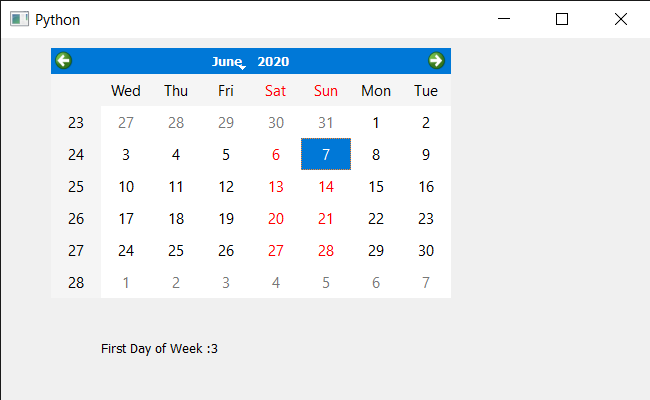# PyQt5 QCalendarWidget – Getting First Day of Week

• Last Updated : 11 Aug, 2021

In this article we will see how we can get the first day of the week of the QCalendarWidget. When we create a calendar by default first day of the week is Monday although we can change this any time, days are displayed in the first column of the calendar. We can change the first day any time with the help of setFirstDayOfWeek method.

In order to do this we will use firstDayOfWeek method with the QCalendarWidget object.

Syntax : calendar.firstDayOfWeek()
Argument : It takes no argument
Return : It return day object but when printed it shows the value associated with them

Below is the implementation

## Python3

 `# importing libraries``from` `PyQt5.QtWidgets ``import` `*``from` `PyQt5 ``import` `QtCore, QtGui``from` `PyQt5.QtGui ``import` `*``from` `PyQt5.QtCore ``import` `*``import` `sys`  `class` `Window(QMainWindow):` `    ``def` `__init__(``self``):``        ``super``().__init__()` `        ``# setting title``        ``self``.setWindowTitle(``"Python "``)` `        ``# setting geometry``        ``self``.setGeometry(``100``, ``100``, ``650``, ``400``)` `        ``# calling method``        ``self``.UiComponents()` `        ``# showing all the widgets``        ``self``.show()` `    ``# method for components``    ``def` `UiComponents(``self``):` `        ``# creating a QCalendarWidget object``        ``self``.calendar ``=` `QCalendarWidget(``self``)` `        ``# setting geometry to the calendar``        ``self``.calendar.setGeometry(``50``, ``10``, ``400``, ``250``)` `        ``# setting cursor``        ``self``.calendar.setCursor(Qt.PointingHandCursor)` `        ``# setting first day of week``        ``self``.calendar.setFirstDayOfWeek(Qt.Wednesday)` `        ``# creating label to show the properties``        ``self``.label ``=` `QLabel(``self``)` `        ``# setting geometry to the label``        ``self``.label.setGeometry(``100``, ``280``, ``250``, ``60``)` `        ``# making label multi line``        ``self``.label.setWordWrap(``True``)`  `        ``# getting first day of the week``        ``value ``=` `self``.calendar.firstDayOfWeek()` `        ``# setting text to the label``        ``self``.label.setText(``"First Day of Week :"` `+` `str``(value))`    `# create pyqt5 app``App ``=` `QApplication(sys.argv)` `# create the instance of our Window``window ``=` `Window()` `# start the app``sys.exit(App.``exec``())`

Output :My Personal Notes arrow_drop_up